The GLMSELECT Procedure

Forward Selection (FORWARD)

The forward selection technique begins with just the intercept and then sequentially adds the effect that most improves the fit. The process terminates when no significant improvement can be obtained by adding any effect.

In the traditional implementation of forward selection, the statistic used to gauge improvement in fit is an F statistic that reflects an effect’s contribution to the model if it is included. At each step, the effect that yields the most significant F statistic is added. Note that because effects can contribute different degrees of freedom to the model, it is necessary to compare the p-values corresponding to these F statistics.

More precisely, if the current model has p parameters excluding the intercept, and if you denote its residual sum of squares by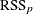and you add an effect with k degrees of freedom and denote the residual sum of squares of the resulting model by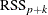, then the F statistic for entry with k numerator degrees of freedom and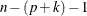denominator degrees of freedom is given by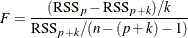where n is number of observations used in the analysis.

The process stops when the significance level for adding any effect is greater than some specified entry significance level. A well-known problem with this methodology is that these F statistics do not follow an F distribution (Draper, Guttman, and Kanemasu, 1971). Hence these p-values cannot reliably be interpreted as probabilities. Various ways to approximate this distribution are described by Miller (2002). Another issue when you use significance levels of entering effects as a stopping criterion arises because the entry significance level is an a priori specification that does not depend on the data. Thus, the same entry significance level can result in overfitting for some data and underfitting for other data.

One approach to address the critical problem of when to stop the selection process is to assess the quality of the models produced by the forward selection method and choose the model from this sequence that "best" balances goodness of fit against model complexity. PROC GLMSELECT supports several criteria that you can use for this purpose. These criteria fall into two groups—information criteria and criteria based on out-of-sample prediction performance.

You use the CHOOSE= option of forward selection to specify the criterion for selecting one model from the sequence of models produced. If you do not specify a CHOOSE= criterion, then the model at the final step is the selected model.

For example, if you specify

selection=forward(select=SL choose=AIC SLE=0.2)


then forward selection terminates at the step where no effect can be added at the 0.2 significance level. However, the selected model is the first one with the minimal value of Akaike’s information criterion. Note that in some cases this minimal value might occur at a step much earlier that the final step, while in other cases the AIC criterion might start increasing only if more steps are done (that is, a larger value of SLE is used). If what you are interested in is minimizing AIC, then too many steps are done in the former case and too few in the latter case. To address this issue, PROC GLMSELECT enables you to specify a stopping criterion with the STOP= option. With a stopping criterion specified, forward selection continues until a local extremum of the stopping criterion in the sequence of models generated is reached. You can also specify STOP= number, which causes forward selection to continue until there are the specified number of effects in the model.

For example, if you specify

selection=forward(select=SL stop=AIC)


then forward selection terminates at the step where the effect to be added at the next step would produce a model with an AIC statistic larger than the AIC statistic of the current model. Note that in most cases, provided that the entry significance level is large enough that the local extremum of the named criterion occurs before the final step, specifying

selection=forward(select=SL choose=CRITERION)


or

selection=forward(select=SL stop=CRITERION)


selects the same model, but more steps are done in the former case. In some cases there might be a better local extremum that cannot be reached if you specify the STOP= option but can be found if you use the CHOOSE= option. Also, you can use the CHOOSE= option in preference to the STOP= option if you want examine how the named criterion behaves as you move beyond the step where the first local minimum of this criterion occurs.

Note that you can specify both the CHOOSE= and STOP= options. You might want to consider models generated by forward selection that have at most some fixed number of effects but select from within this set based on a criterion you specify. For example, specifying

selection=forward(stop=20 choose=ADJRSQ)


requests that forward selection continue until there are 20 effects in the final model and chooses among the sequence of models the one that has the largest value of the adjusted R-square statistic. You can also combine these options to select a model where one of two conditions is met. For example,

selection=forward(stop=AICC choose=PRESS)


chooses whatever occurs first between a local minimum of the predicted residual sum of squares (PRESS) and a local minimum of corrected Akaike’s information criterion (AICC).

It is important to keep in mind that forward selection bases the decision about what effect to add at any step by considering models that differ by one effect from the current model. This search paradigm cannot guarantee reaching a "best" subset model. Furthermore, the add decision is greedy in the sense that the effect deemed most significant is the effect that is added. However, if your goal is to find a model that is best in terms of some selection criterion other than the significance level of the entering effect, then even this one step choice might not be optimal. For example, the effect you would add to get a model with the smallest value of the PRESS statistic at the next step is not necessarily the same effect that has the most significant entry F statistic. PROC GLMSELECT enables you to specify the criterion to optimize at each step by using the SELECT= option. For example,

selection=forward(select=CP)


requests that at each step the effect that is added be the one that gives a model with the smallest value of the Mallows’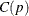statistic. Note that in the case where all effects are variables (that is, effects with one degree of freedom and no hierarchy), using ADJRSQ, AIC, AICC, BIC, CP, RSQUARE, or SBC as the selection criterion for forward selection produces the same sequence of additions. However, if the degrees of freedom contributed by different effects are not constant, or if an out-of-sample prediction-based criterion is used, then different sequences of additions might be obtained.

You can use SELECT= together with CHOOSE= and STOP= . If you specify only the SELECT= criterion, then this criterion is also used as the stopping criterion. In the previous example where only the selection criterion is specified, not only do effects enter based on the Mallows’statistic, but the selection terminates when thestatistic first increases.

You can find discussion and references to studies about criteria for variable selection in Burnham and Anderson (2002), along with some cautions and recommendations.

Examples of Forward Selection Specifications
selection=forward


adds effects that at each step give the lowest value of the SBC statistic and stops at the step where adding any effect would increase the SBC statistic.

selection=forward(select=SL)


adds effects based on significance level and stops when all candidate effects for entry at a step have a significance level greater than the default entry significance level of 0.15.

selection=forward(select=SL stop=validation)


adds effects based on significance level and stops at a step where adding any effect increases the error sum of squares computed on the validation data.

selection=forward(select=AIC)


adds effects that at each step give the lowest value of the AIC statistic and stops at the step where adding any effect would increase the AIC statistic.

selection=forward(select=ADJRSQ stop=SL SLE=0.2)


adds effects that at each step give the largest value of the adjusted R-square statistic and stops at the step where the significance level corresponding to the addition of this effect is greater than 0.2.